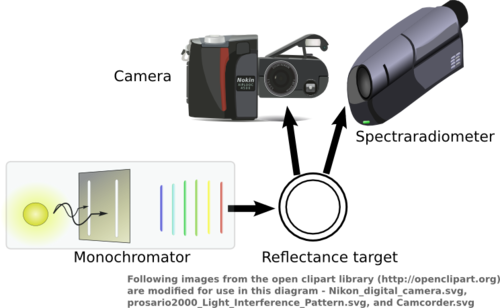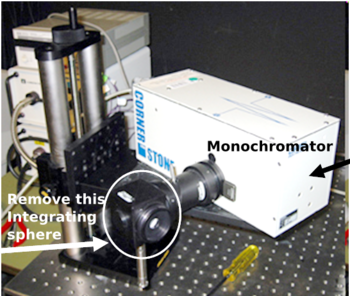#  Multispectral imaging

Multispectral imaging involves measuring the wavelength-dependent distribution of energy at imaged points in a scene. Sometimes, we will know the spectral power distribution (SPD) of the ambient illumination; then, we try to estimate the spectral reflectance functions of the scene points. In color digital imaging applications, usually SPDs are digitized by sampling them at every 10 nm in the wavelength interval [400,700] nm (roughly the visible range of the spectrum) and we need to measure the values of the SPD at 31 different wavelengths.

Commonly, special instruments designed to measure SPDs (spectraphotometers or spectraradiometers) make measurements that are averaged over a large number of points in the scene. To acquire 2D multispectral imgage data, a common techinque is to use a digital camera (color or monochrome) augmented in some way.

Digital color cameras usually give three readings. These are three differently weighted sums of the SPDs of scene points. The three sets of weights have dominating values in different regions of the spectrum, typically, red, green, and blue. Without augmenting these three measurements in some way, we would need to estimate at each point 31 values from only three measurements. This is usually a difficult task. Instead, it is common to take multiple acquisitions with a color camera to get more measurements. The multiple measurements are taken either with different optical filters or with different illuminants. An optical filter or an illuminant alter the weights associated with the summed SPDs and allow us to increase the number of measurements. For example, we may acquire three different images with a color camera under different illuminant combinations to make a total of 9 measurements. Then, we only have to estimate 31 values from 9 measurements. This is usually possible since common SPDs vary slowly with wavelength.

Mathematically, for each point in the scene we have to solve the problem:

$y = S^T x\,,$

where $S$ has size $31 \times n$. $n$ is the number of measurements and each column of $S$ has the weights associated with a camera color filter and illuminant (or optical filter) combination. There are several ways to address this problem. Some of them are described in the Reflectance and Illuminant Estimation page.

#  Experiments - data acquisition

Our multispectral imaging setup is built around the LED-based illuminator Max Klein designed and built for his Psych 221 project (Winter 2008). The specifications of this illuminator and details about its design and construction (including CAD diagrams and Spice models) are online at his project webpage. Here we describe its use to acquire multispectral images. We need the following equipment:

• A calibrated camera
• The multispectral illuminator and its controller (hyperterminal on a PC)
• A uniform gray chart for spatial calibration

##  Camera calibration

During camera calibration we determine the spectral sensitivity functions of the camera sensor. These functions describe the wavelength-dependent weights associated with the camera color channels. We can find these weights by relating camera measurements of a number of known monochromatic lights to their corresponding SPD measurements. We solve the following mathematical problem to find $S$:

$Y = S^T X\,,$

where we measure $k$ different lights with the camera and a spectraradiometer. $Y$ is of size $3 \times k$ and its columns hold the camera measurements. The columns of $X$ hold the corresponding SPD measurements.

Figure 1 shows a diagram of how the different devices are set up. The monochromator is the light source and is capable of producing light in several different narrowband ranges of wavelengths. The light from the monochromator is made to fall on a standard reflectance target. The spectraradiometer measures the SPD of the light, while the camera to be calibrated takes an image of the same light. We collect measurements of SPDs and corresponding camera images to find $S$.Figure 1: Camera calibration A monochromator (the ORIEL 3000) is used to shine several narrowband lights onto a reflectance target. The monochromatic light is measured simultaneously with the camera and a spectraradiometer (the PR-650 or the PR-715).Figure 2: Remove the integrating sphere We need to remove the integrating sphere that is typically attached to the monochromator. Otherwise, the intensity of light is very low and we can not acquire images in reasonable lengths of time.